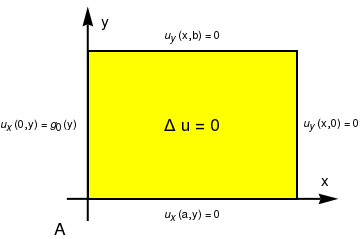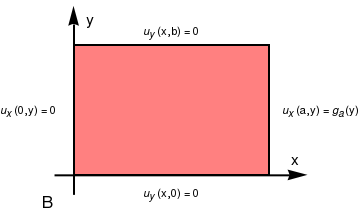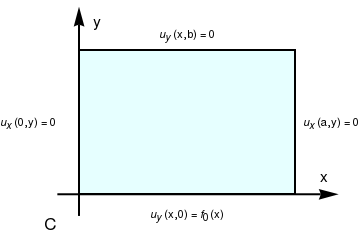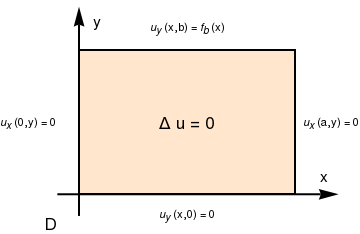# Preface

This section presents examples of solving Neumann boundary value problems for the Laplace and Helmholtz equations in rectangular coordinates.

Introduction to Linear Algebra with Mathematica

# Neumann Problems for Laplace Equation

Let Ω be a domain in ℝn have a smooth boundary ∂Ω; then the outer unit normal vector n is defined for every point on the boundary ∂Ω. The Neumann boundary conditions,
$\left. \frac{\partial u}{\partial{\bf n}} \right\vert_{x\in \partial\Omega} = f(x)$
are imposed on a function u(x) defined inside of Ω; here f(x) is a specified function on the boundary ∂Ω. This boundary conditions are named after Carl Neumann (1832–1925), a German mathematician who worked on infinite series and developed an early model of electromagnetism.
The problem of finding a function u(x) harmonic inside a region Ω (Δu = 0) and whose normal derivative takes given values on its boundary ∂Ω is called the interior Neumann problem or second boundary value problem (inner) for Ω. If a harmonic function is seeking outside the region Ω, then we have the exterior Neumann problem.

Laplace's equation is a second-order partial differential equation named after Pierre-Simon Laplace who, beginning in 1782, studied its properties while investigating the gravitational attraction of arbitrary bodies in space. However, the equation first appeared in 1752 in a paper by Euler on hydrodynamics. Laplace's equation is often written as:

\begin{equation} \label{EqNeumann.1} \Delta u ({\bf x}) =0 \qquad \mbox{or} \qquad \frac{\partial^2 u}{\partial x_1^2} + \frac{\partial^2 u}{\partial x_2^2} + \cdots +\frac{\partial^2 u}{\partial x_n^2} =0 \qquad \mbox{in domain } {\bf x} \in \Omega \subset \mathbb{R}^n , \end{equation}
where $$\Delta = \nabla^2 = \nabla \cdot \nabla$$ is the Laplace operator or Laplacian. Another very important version of Eq.\eqref{EqNeumann.1} is the so called the Helmholtz equation
\begin{equation} \label{EqNeumann.2} \Delta u ({\bf x}) + k^2 u =0 \qquad \mbox{in domain } {\bf x} \in \Omega \subset \mathbb{R}^n , \end{equation}
named for the German physician and physicist Hermann von Helmholtz (1821--1894). A nonhomogeneous version of the Laplace equation
\begin{equation} \label{EqNeumann.3} \Delta u ({\bf x}) = f({\bf x}) \qquad \mbox{in domain } \Omega \subset \mathbb{R}^n , \end{equation}
where f is a given smooth function, is called the Poisson's equation. The equation is named after the French mathematician, geometer, and physicist Siméon Denis Poisson (1781--1840). In two dimensions, the Laplace equation in rectangular coordinates becomes
$\frac{\partial^2 u}{\partial x^2} + \frac{\partial^2 u}{\partial y^2} =0 \qquad\mbox{or for short} \qquad u_{xx} + u_{yy} =0 .$
Correspondingly, in three dimensions, the Poisson's equation is
$u_{xx} + u_{yy} + u_{zz} = f(x,y,z) ,$
where f is a given smooth function. The Laplacian occurs in differential equations that describe many physical phenomena, such as electric and gravitational potentials, the diffusion equation for heat and fluid flow, wave propagation, and quantum mechanics. The Laplacian represents the flux density of the gradient flow of a function. For instance, the net rate at which a chemical dissolved in a fluid moves toward or away from some point is proportional to the Laplacian of the chemical concentration at that point.

Since there is no time dependence in the Laplace's equation or Poisson's equation, there is no initial conditions to be satisfied by their solutions. However, there should be certain boundary conditions on the boundary curve or surface $$\partial\Omega$$ of the region Ω in which the differential equation is to be solved. Typically, there are three types of boundary conditions.

The problem of finding a solution of Laplace's equation that takes on given boundary values is known as a Dirichlet problem. On the other hand, if the values of the normal derivative are prescribed on the boundary, the problem is said to be a Neumann problem:
\begin{align*} &\text{Laplace's equation:} \qquad &u_{xx} + u_{yy} &= 0 , \quad (x,y) \in \Omega \subset \mathbb{R}^2 , \\ &\text{Neumann boundary condition:} \hspace{6.2mm} &\left. \frac{\partial u}{\partial {\bf n}} \right\vert_{(x,y) \in \partial\Omega} &= f(P), \quad P\in \partial\Omega , \quad {\bf n} \mbox{ is a unit outward normal vector to the boundary } \partial\Omega , \\ &\text{Solvability condition:} \qquad &\int_{\partial\Omega} f(P)\,{\text d}S_p &= 0 . \end{align*}
Here f(P) is a prescribed function defined on the smooth boundary ∂Ω of the domain Ω; its integral over the boundary must be zero, otherwise, the Neumann boundary value problem has no solution.

Physically, it is plausible to expect that three types of boundary conditions will be sufficient to determine the solution completely. Indeed, it is possible to establish the existence and uniqueness of the solution of Laplace's (and Poisson's) equation under the first and third type boundary conditions, provided that the boundary $$\partial\Omega$$ of the domain Ω is smooth (have no corner or edge). However, we avoid explicit statements and their proofs because the material is beyond the scope of the tutorial. Instead, we concentrate our attention on some typical problems that could be solved by means of separation of variables.

Neumann Problem for a Rectangle

The general interior Neumann problem for Laplace's equation in rectangular domain $$[0,a] \times [0,b]$$ using Cartesian coordinates can be formulated as follows. Find the solution of Laplace's equation
\begin{equation} \label{EqNeumann.4} \Delta u \equiv u_{xx} + u_{yy} =0 , \qquad 0 < x < a, \quad 0< y <b ; \end{equation}
subject to the boundary conditions of the second kind
\begin{equation} \label{EqNeumann.5} \left. \frac{\partial u}{\partial x} \right\vert_{x=0} = u_x (0,y) = g_0 (y) , \qquad \left. \frac{\partial u}{\partial x} \right\vert_{x=a} = u_x (a,y) = g_a (y) , \qquad 0 < y < b, \end{equation}
and
\begin{equation} \label{EqNeumann.6} \left. \frac{\partial u}{\partial y} \right\vert_{y=0} = u_y (x,0) = f_0 (x) , \qquad \left. \frac{\partial u}{\partial y} \right\vert_{y=b} = u_y (x,b) = f_b (x) , \qquad 0 < x < a ; \end{equation}
where f0, fb, g0, and ga are given functions. Here we use the shortcut notation ux and uy for partial derivatives with respect to x and y, respectively.

Using superposition principle, we can break the given Neumann problems into four similar problems when flux source comes only from one side of the rectangle, and the other three sides are isolated. Hence, we can represent the solution as the sum of four functions

$u(x,y) = u_A (x,y) + u_B (x,y) + u_C (x,y) + u_D (x,y) ,$
Each function in the right-hand side is a solution of Laplace's equation with insulated three sides. Therefore, we get four Neumann problems. (Be aware that your browser may not display the codes properly.) Note that problems A and B could be united into one, as well as problems C and D.
rectangle = Graphics[{EdgeForm[Thick], Yellow, Rectangle[{0, 0}, {3, 2}]}];
aA = Graphics[Text[Style["A", FontSize -> 16], {-0.4, -0.4}]];
a0 = Graphics[ Text[Style[ "\!$$\*SubscriptBox[\(u$$, $$x$$]\)(a,y) = 0"], {1.5, -0.2}]];
a1 = Graphics[ Text[Style["\!$$\*SubscriptBox[\(u$$, $$y$$]\)(x,0) = 0"], {3.5, 1.0}]];
a2 = Graphics[ Text[Style["\!$$\*SubscriptBox[\(u$$, $$y$$]\)(x,b) = 0"], {1.5, 2.2}]];
ax = {Arrowheads[Large], Arrow[{{-0.3, 0}, {3.6, 0}}]};
ax1 = Graphics[{Thick, Black, ax}];
ay = {Arrowheads[Large], Arrow[{{0, -0.3}, {0, 2.6}}]};
ay1 = Graphics[{Thick, Black, ay}];
txt1 = Graphics[Text[Style["x", FontSize -> 14], {3.4, 0.25}]];
txt2 = Graphics[Text[Style["y", FontSize -> 14], {0.25, 2.4}]];
left = Graphics[ Text[Style[ "\!$$\*SubscriptBox[\(u$$, $$x$$]\)(0,y) = \ \!$$\*SubscriptBox[\(g$$, $$0$$]\)(y)"], {-0.7, 1}]];
delta = Graphics[ Text[Style["$CapitalDelta] u = 0", FontSize -> 16], {1.5, 1.0}]]; Show[ax1, ay1, rectangle, txt1, txt2, delta, left, a0, a1, a2, aA, AspectRatio -> 3/4] rectangleB = Graphics[{EdgeForm[Thick], Pink, Rectangle[{0, 0}, {3, 2}]}]; right = Graphics[ Text[Style[ "\!$$\*SubscriptBox[\(u$$, $$x$$]\)(a,y) = \ \!$$\*SubscriptBox[\(g$$, $$a$$]\)(y)"], {3.8, 1}]]; a0 = Graphics[ Text[Style["\!$$\*SubscriptBox[\(u$$, $$x$$]\)(0,y) = 0"], {-0.7, 1.0}]]; a1 = Graphics[ Text[Style[ "\!$$\*SubscriptBox[\(u$$, $$y$$]\)(x,0) = 0"], {1.5, -0.3}]]; a2 = Graphics[ Text[Style["\!$$\*SubscriptBox[\(u$$, $$y$$]\)(x,b) = 0"], {1.5, 2.2}]]; aB = Graphics[Text[Style["B", FontSize -> 16], {-0.4, -0.4}]]; Show[rectangleB, right, txt1, txt2, ax1, ay1, a0, a1, a2, aB] rectangleC = Graphics[{EdgeForm[Thick], LightCyan, Rectangle[{0, 0}, {3, 2}]}]; down = Graphics[ Text[Style[ "\!$$\*SubscriptBox[\(u$$, $$y$$]\)(x,0) = \ \!$$\*SubscriptBox[\(f$$, $$0$$]\)(x)"], {1.5, -0.3}]]; a0 = Graphics[ Text[Style["\!$$\*SubscriptBox[\(u$$, $$x$$]\)(0,y) = 0"], {-0.7, 1.0}]]; a1 = Graphics[ Text[Style["\!$$\*SubscriptBox[\(u$$, $$x$$]\)(a,y) = 0"], {3.5, 1.0}]]; a2 = Graphics[ Text[Style["\!$$\*SubscriptBox[\(u$$, $$y$$]\)(x,b) = 0"], {1.5, 2.2}]]; aC = Graphics[Text[Style["C", FontSize -> 16], {-0.4, -0.4}]]; Show[rectangleC, down, txt1, txt2, ax1, ay1, a0, a1, a2, aC] rectangleD = Graphics[{EdgeForm[Thick], LightOrange, Rectangle[{0, 0}, {3, 2}]}]; top = Graphics[ Text[Style[ "\!$$\*SubscriptBox[\(u$$, $$y$$]\)(x,b) = \ \!$$\*SubscriptBox[\(f$$, $$b$$]\)(x)"], {1.5, 2.3}]]; a0 = Graphics[ Text[Style["\!$$\*SubscriptBox[\(u$$, $$x$$]\)(0,y) = 0"], {-0.7, 1.0}]]; a1 = Graphics[ Text[Style["\!$$\*SubscriptBox[\(u$$, $$x$$]\)(a,y) = 0"], {3.5, 1.0}]]; a2 = Graphics[ Text[Style[ "\!$$\*SubscriptBox[\(u$$, $$y$$]\)(x,0) = 0"], {1.5, -0.3}]]; aD = Graphics[Text[Style["D", FontSize -> 16], {-0.4, -0.4}]]; Show[rectangleD, top, txt1, txt2, ax1, ay1, a0, a1, a2, aD, delta]Problem 1A: consists of the partial differential equation $$\Delta u =0$$ in the rectangle $$(0,a) \times (0,b)$$ subject to the boundary conditions: \[ \left. \frac{\partial u}{\partial x} \right\vert_{x=0} = u_x (0,y) = g_0 (y) , \qquad \left. \frac{\partial u}{\partial x} \right\vert_{x=a} = u_x (a,y) = 0, \qquad 0 < y < b;\qquad \left. \frac{\partial u}{\partial y} \right\vert_{y=0} = u_y (x,0) = 0 , \qquad \left. \frac{\partial u}{\partial y} \right\vert_{y=b} = u_y (x,b) = 0 .$
To solve problem A, we proceed in exactly the same as in the previous problem: set $$u(x,y) = X(x)\,Y(y)$$ and substitute into the Laplace equation and homogeneous boundary conditions in y. This give the familiar Sturm--Liouville problem for Y:
$Y'' + \lambda\,Y =0 , \qquad Y'(0) =0, \quad Y'(b) =0.$
Its solution is well-known:
$\lambda_n = \left( \frac{n\pi}{b} \right)^2 , \qquad Y_n (y) = \cos \frac{n\pi y}{b} , \qquad n=0,1,2,\ldots .$
Note that zero is an eigenvalue to which corresponds a constant eigenfunction. For X, we get the following differential equation:
$X'' (x) - \left( \frac{n\pi}{b} \right)^2 X(x) =0 ,$
which has the general solution
$X_n (x) = c_{1n} \cosh \frac{n\pi x}{b} + c_{2n} \sinh \frac{n\pi x}{b} .$
The solution of the given Neumann problem A for Laplace's equation is assumed to be represented as the sum of all partial nontrivial solutions:
$u(x,y) = C+\sum_{n\ge 1} X_n (x)\, Y_n (y) ,$
where C is an arbitrary constant. Since each term in the above sum satisfies the homogeneous boundary conditions $$u_y (x,0) = u_y (x,b) =0 ,$$ we know that the sum has the same property (subject to uniform convergence, which is assumed). To satisfy the boundary conditions in variable x, we have to consider two equations
$u_x (0,y) = \sum_{n\ge 1} X'_n (0)\, Y_n (y) = g_0 (y) \qquad\mbox{and} \qquad u_x (a,y) = \sum_{n\ge 1} X'_n (a)\, Y_n (y) = 0 .$

Problem 1B: consists of the partial differential equation $$\Delta u =0$$ in the rectangle $$(0,a) \times (0,b)$$ subject to the boundary conditions:

$\left. \frac{\partial u}{\partial x} \right\vert_{x=0} = u_x (0,y) = 0 , \qquad \left. \frac{\partial u}{\partial x} \right\vert_{x=a} = u_x (a,y) = g_a (y) ,\qquad 0 < y < b;\qquad \left. \frac{\partial u}{\partial y} \right\vert_{y=0} = u_y (x,0) = 0 , \qquad \left. \frac{\partial u}{\partial y} \right\vert_{y=b} = u_y (x,b) = 0 .$
Before solving the given boundary value problem, you need to check whether solvability condition holds:
$\int_0^b g_a (y)\,{\text d}y = 0.$
If this integral is not zero, the problem has no solution. Remember that if the interior Neumann problem has a solution, it is not unique and arbitrary constant can be added.

Note that it has homogeneous boundary conditions in variable y and one homogeneous boundary condition at x = 0. To solve problem B, we proceed in exactly the same as in the previous problem: set $$u(x,y) = X(x)\,Y(y)$$ and substitute into the Laplace equation and homogeneous boundary conditions in variable y. This give the familiar Sturm--Liouville problem for Y:

$Y'' + \lambda\,Y =0 , \qquad Y'(0) =0, \quad Y'(b) =0.$
Its solution is well-known:
$\lambda_n = \left( \frac{n\pi}{b} \right)^2 , \qquad Y_n (y) = \cos \frac{n\pi y}{b} , \qquad n=0,1,2,\ldots .$
Note that zero is an eigenvalue to which corresponds a constant eigenfunction. For X, we get the following differential equation:
$X'' (x) - \left( \frac{n\pi}{b} \right)^2 X(x) =0 ,$
which has the general solution
$X_n (x) = c_{1n} \cosh \frac{n\pi x}{b} + c_{2n} \sinh \frac{n\pi x}{b} .$
The solution of the given Neumann problem B for Laplace's equation is assumed to be represented as the sum of all partial nontrivial solutions (subject that the series converges):
$u(x,y) = C+\sum_{n\ge 1} X_n (x)\, Y_n (y) ,$
where C is an arbitrary constant. Since each term in the above sum satisfies the homogeneous boundary conditions $$u_y (x,0) = u_y (x,b) =0 ,$$ we know that the sum has the same property (subject to uniform convergence, which is assumed). To satisfy the boundary conditions in variable x, we have to consider two equations
$u_x (0,y) = \sum_{n\ge 1} X'_n (0)\, Y_n (y) =0 \qquad\mbox{and} \qquad u_x (a,y) = \sum_{n\ge 1} X'_n (a)\, Y_n (y) = g_a (y) .$
Therefore, if we set $$X'_n (0) =0 ,$$ which is equivalent to $$c_{2n} =0,$$ it will guarantee that the first boundary condition holds. With this in hand, we obtain the general solution:
$u(x,y) = C+\sum_{n\ge 1} X_n (x)\, Y_n (y) = C+\sum_{n\ge 1} A_n \cosh \frac{n\pi x}{b} \, \cos \frac{n\pi y}{b} ,$
where coefficients An should be chosen so that the identity
$\left. \frac{\partial u}{\partial x} \right\vert_{x=a} = \sum_{n\ge 1} X'_n (a)\, Y_n (y) = \sum_{n\ge 1} A_n \,\frac{n\pi }{b} \, \sinh \frac{n\pi a}{b} \, \cos \frac{n\pi y}{b} = g_a (y) .$
is valid. However, this is just Fourier cosine series for function g, and its coefficients follow from Euler's formulas:
$A_n \,\frac{n\pi }{b} \, \sinh \frac{n\pi a}{b} = \frac{2}{b} \int_0^b g_a (y)\, \cos \frac{n\pi y}{b} \,{\text d}y \qquad \Longrightarrow \qquad A_n = \dfrac{2}{n\pi \, \sinh \frac{n\pi a}{b}} \, \int_0^b g_a (y)\, \cos \frac{n\pi y}{b} \,{\text d}y , \qquad n=1,2,3,\ldots .$
Since the constant term in cosine Fourier series is absent, the expansion above is valid only when
$\int_0^b g_a (y)\,{\text d}y =0.$
In this case, the Neumann problem has a solution up to arbitrary constant, so it is not unique:
$u(x,y) = C + \sum_{n\ge 1} \cos \frac{n\pi y}{b} \cdot \dfrac{2\,\cosh \frac{n\pi x}{b}}{n\pi \, \sinh \frac{n\pi a}{b}} \, \int_0^b g_a (y)\, \cos \frac{n\pi y}{b} \,{\text d}y .$
■

Problem 1C: consists of the partial differential equation $$\Delta u =0$$ in the rectangle $$(0,a) \times (0,b)$$ subject to the boundary conditions:

$\left. \frac{\partial u}{\partial x} \right\vert_{x=0} = u_x (0,y) = 0 , \qquad \left. \frac{\partial u}{\partial x} \right\vert_{x=a} = u_x (a,y) = 0, \qquad 0 < y < b;\qquad \left. \frac{\partial u}{\partial y} \right\vert_{y=0} = u_y (x,0) = f_0 (x) , \qquad \left. \frac{\partial u}{\partial y} \right\vert_{y=b} = u_y (x,b) = 0 , \quad 0 < x < b.$
■

Problem 1D: consists of the partial differential equation $$\Delta u =0$$ in the rectangle $$(0,a) \times (0,b)$$ subject to the boundary conditions:

$\left. \frac{\partial u}{\partial x} \right\vert_{x=0} = u_x (0,y) = 0 , \qquad \left. \frac{\partial u}{\partial x} \right\vert_{x=a} = u_x (a,y) = 0, \qquad 0 < y < b;\qquad \left. \frac{\partial u}{\partial y} \right\vert_{y=0} = u_y (x,0) = 0 , \qquad \left. \frac{\partial u}{\partial y} \right\vert_{y=b} = u_y (x,b) = f_b (x) , \quad 0 < x < a .$
■

1. Venttsel’, A.D., On boundary conditions for multi-dimensional diffusion processes (in Russian), Teoriya Veroyatnostei i ee Primeneniya, 4, 172-185, English Translation: Theory of Probability & Its Applications, 1959, Vol. 4, Issue 2, pp. 164–177; https://doi.org/10.1137/1104014